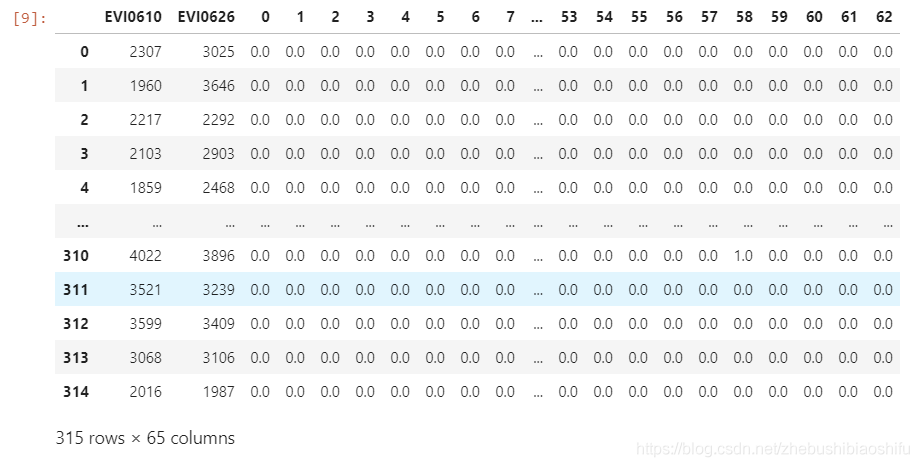# Python實現類別變量的獨熱編碼

## 1 OneHotEncoder

```import pandas as pd
from sklearn.preprocessing import OneHotEncoder
```

```test_data_1=pd.read_csv('G:/CropYield/03_DL/00_Data/onehot_test.csv',names=['EVI0610','EVI0626','SoilType'],header=0)
``````ohe=OneHotEncoder(handle_unknown='ignore')
ohe.fit(test_data_1)
``````ohe.categories_
``````ohe_column=pd.DataFrame(ohe.fit_transform(test_data_1[['SoilType']]).toarray())
``````count=pd.DataFrame(test_data_1['SoilType'].value_counts())
print(count)
``````test_data_1.head(5)
``````test_data_1=test_data_1.drop(['SoilType'],axis=1)
``````test_data_1.join(ohe_column)
```## 2 pd.get_dummies

`pd.get_dummies`是一個最好的辦法！其具體用法與上述`OneHotEncoder`類似，因此具體過程就不再贅述啦，大傢看代碼就可以明白。

```test_data_2=pd.read_csv('G:/CropYield/03_DL/00_Data/onehot_test.csv',names=['EVI0610','EVI0626','SoilType'],header=0)
``````test_data_2_ohe=pd.get_dummies(test_data_2,columns=['SoilType'])